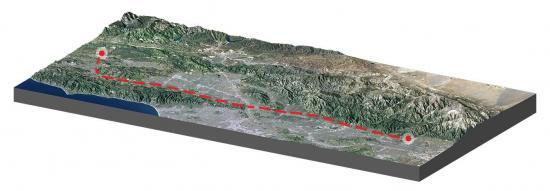Home » GIS Analysis » Least Cost Path Analysis in GIS

# Least Cost Path Analysis in GIS### How Does the Least Cost Path Work?

The least cost path finds the most cost-effective path, from a start point to a destination.

As it goes from start to finish, the chosen path accumulates the least amount of “cost”.

This analysis is very practical in linear infrastructure and routing applications.

For example, pipeline, powerline, and trail planning heavily rely on this type of analysis for route selection.

### Examples and Applications

Some of the areas where this raster analysis is used in archaeology, animal corridors, and engineering.

Here are two in-depth examples of least cost path and how it works:

Pipeline Routing – If you want to find the most cost-effective route for a pipeline, you’d likely want to design it where it costs the least. For example, the cost distance layer would include weighted values from population density, environmental sites, and proximity to water. Impediments would be anything you can’t cross like cemeteries, restricted areas, or sacred sites.

Hiking Trail Planning – The “cost” would be very different for planning a family-friendly hiking trail. In this case, you would want to build it on the least amount of slope so it’s safe to climb. As you go from the start point to its destination, you want to “buy” the lowest “cost”, which would be the least amount of slope. Any route to the top equals the same net change in elevation. But the least cost path accumulates the least amount of slope from start to finish.

### How To Use the Least Cost Path

There are three main steps when you run this analysis:

• Cost distance – First, you have to calculate the cost in relation to your start point. For routing a hiking trail, your cost matrix would be the slope. The higher the slope, the more costly it would be.
• Cost backlink – Second, you need to calculate the raster backlink. In this case, it would be the direction in which the path will go with all the 8 possible cardinal directions from one cell to the next.
• Cost path – Finally, you can calculate the least cost path using the cost distance, cost backlink, and destination source. No matter which route, any path to the destination equals the same net change in elevation. But the least cost path accumulates the least amount of slope.

The result is the most cost-effective route from the starting point to the final destination.

### Summary

The least cost path may be one of the best tools in the GIS arsenal for any linear infrastructure and routing application.

But the key is that your input data are all logical, accurate, and complete.

Only then can it determine the most cost-effective path by accumulating the least amount of “cost”.

Are you try to find more raster analysis tools. Then, here are some more that can help you become a raster master: